Updating search results...

# 27 Results

View
Selected filters:
• NC.Math.8.NS.2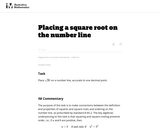Unrestricted Use
CC BY
Rating
0.0 stars

This is a task from the Illustrative Mathematics website that is one part of a complete illustration of the standard to which it is aligned. Each task has at least one solution and some commentary that addresses important asects of the task and its potential use. Here are the first few lines of the commentary for this task: Place $\sqrt{28}$ on a number line, accurate to one decimal point....

Subject:
Mathematics
Material Type:
Activity/Lab
Provider:
Illustrative Mathematics
Provider Set:
Illustrative Mathematics
Author:
Illustrative Mathematics
05/29/2013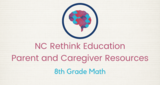Conditional Remix & Share Permitted
CC BY-NC-SA
Rating
0.0 stars

This 8th grade Math&nbsp;parent guide&nbsp;explains the content in straightforward terms so they can support their children&rsquo;s learning at home and will&nbsp;encourage&nbsp;caretaker engagement&nbsp;with lessons.

Subject:
Mathematics
Material Type:
Reference Material
Author:
Kelly Rawlston
Letoria Lewis
01/10/2022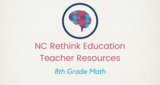Conditional Remix & Share Permitted
CC BY-NC-SA
Rating
0.0 stars

Our Teacher Guides are meant to support the use of our online course and unit content. Please use these to accompany the use of our content and for ideas to support struggling learners, those needing extension and for additional resources.

Subject:
Mathematics
Material Type:
Curriculum
Teaching/Learning Strategy
Author:
Kelly Rawlston
Letoria Lewis
05/16/2022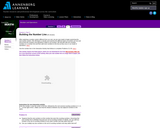Rating
0.0 stars

Students classify the different types of numbers we use, and learn how numbers and operations relate to one another. They start with counting numbers and then add integers, rationals, algebraic, and, finally, real numbers to the line.

Subject:
Mathematics
Material Type:
Activity/Lab
Provider:
Annenberg Learner
Author:
Annenberg Learner
02/26/2019Conditional Remix & Share Permitted
CC BY-NC
Rating
0.0 stars

In CK-12 Middle School Math Concepts Grade 8, the learning content is divided into concepts. Each concept is complete and whole providing focused learning on an indicated objective. Theme-based concepts provide students with experiences that integrate the content of each concept. Students are given opportunities to practice the skills of each concept through real-world situations, examples, guided practice and explore more practice. There are also video links provided to give students an audio/visual way of connecting with the content.

Subject:
Mathematics
Material Type:
Full Course
Lesson Plan
Textbook
Unit of Study
Provider:
CK-12 Foundation
Provider Set:
CK-12 FlexBook
12/06/2018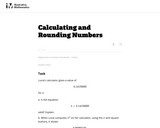Unrestricted Use
CC BY
Rating
0.0 stars

This task is intended for instructional (rather than assessment) purposes, providing an opportunity to discuss technology as it relates to irrational numbers and calculations in general. The task gives a concrete example where rounding and then multiplying does not yield the same answer as multiplying and then rounding.

Subject:
Mathematics
Material Type:
Activity/Lab
Provider:
Illustrative Mathematics
Provider Set:
Illustrative Mathematics
Author:
Illustrative Mathematics
09/13/2012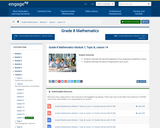Conditional Remix & Share Permitted
CC BY-NC-SA
Rating
0.0 stars

For this lesson, students calculate the decimal expansion of pi using basic properties of area. Students estimate the value of expressions such as pi2.

Subject:
Mathematics
Material Type:
Lesson Plan
Provider:
EngageNY
02/24/2017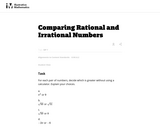Unrestricted Use
CC BY
Rating
0.0 stars

This task can be used to either build or assess initial understandings related to rational approximations of irrational numbers.

Subject:
Mathematics
Material Type:
Activity/Lab
Provider:
Illustrative Mathematics
Provider Set:
Illustrative Mathematics
Author:
Illustrative Mathematics
05/01/2012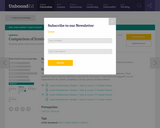Conditional Remix & Share Permitted
CC BY-NC-SA
Rating
0.0 stars

In this lesson, students place irrational numbers in their approximate locations on a number line.

Subject:
Mathematics
Material Type:
Lesson Plan
Provider:
Unbound Ed
Author:
Unbound Ed
04/23/2019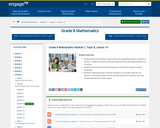Conditional Remix & Share Permitted
CC BY-NC-SA
Rating
0.0 stars

For this lesson, students know the intuitive reason why every repeating decimal is equal to a fraction. Students convert a decimal expansion that eventually repeats into a fraction. Students know that the decimal expansions of rational numbers repeat eventually. Students understand that irrational numbers are numbers that are not rational. Irrational numbers cannot be represented as a fraction and have infinite decimals that never repeat.

Subject:
Mathematics
Material Type:
Lesson Plan
Provider:
EngageNY
02/24/2017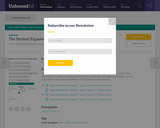Educational Use
Rating
0.0 stars

In this lesson, students calculate the decimal expansion of ? using basic properties of area.

Subject:
Mathematics
Material Type:
Lesson Plan
Provider:
Unbound Ed
Author:
Unbound Ed
04/23/2019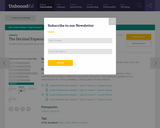Educational Use
Rating
0.0 stars

In this lesson, students use rational approximation to get the approximate decimal expansion of numbers like ?3 and ?28.

Subject:
Mathematics
Material Type:
Lesson Plan
Provider:
Unbound Ed
Author:
Unbound Ed
04/23/2019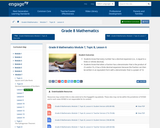Conditional Remix & Share Permitted
CC BY-NC-SA
Rating
0.0 stars

Students know that every number has a decimal expansion (i.e., is equal to a finite or infinite decimal). Students know that when a fraction has a denominator that is the product of 2’s and/or 5’s, it has a finite decimal expansion because the fraction can then be written in an equivalent form with a denominator that is a power of 10.

Subject:
Mathematics
Material Type:
Lesson Plan
Provider:
EngageNY
02/24/2017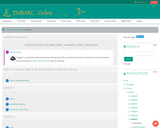Conditional Remix & Share Permitted
CC BY-NC-SA
Rating
0.0 stars

Grade 8 Module 7: Introduction to Irrational Numbers Using Geometry. Contains 23 Lessons.

Subject:
Mathematics
Material Type:
Module
Provider:
EMBARC.Online
08/13/2019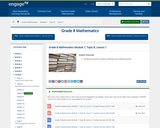Conditional Remix & Share Permitted
CC BY-NC-SA
Rating
0.0 stars

For this lesson, students know the intuitive meaning of an infinite decimal.

Subject:
Mathematics
Material Type:
Lesson Plan
Provider:
EngageNY
02/24/2017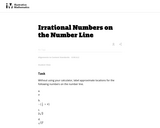Unrestricted Use
CC BY
Rating
0.0 stars

When students plot irrational numbers on the number line, it helps reinforce the idea that they fit into a number system that includes the more familiar integer and rational numbers.

Subject:
Mathematics
Material Type:
Activity/Lab
Provider:
Illustrative Mathematics
Provider Set:
Illustrative Mathematics
Author:
Illustrative Mathematics
05/01/2012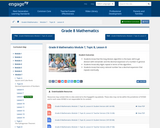Conditional Remix & Share Permitted
CC BY-NC-SA
Rating
0.0 stars

For this lesson, students know that the long division algorithm is the basic skill to get division-with-remainder and the decimal expansion of a number in general. Students know why digits repeat in terms of the algorithm. Students know that every rational number has a decimal expansion that repeats eventually.

Subject:
Mathematics
Material Type:
Lesson Plan
Provider:
EngageNY
02/24/2017Educational Use
Rating
0.0 stars

With this assignment, students locate and identify irrational numbers at the approximate location on a number line. Students will also compare and/or order any real numbers (rational and irrational may be mixed).

Subject:
Mathematics
Material Type:
Homework/Assignment
Provider:
Woodland Hills School District
Author:
Woodland Hills School District
04/23/2019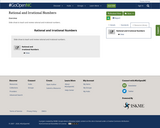Unrestricted Use
CC BY
Rating
0.0 stars

Slide show to teach and review rational and irrational numbers.

Subject:
Mathematics
Material Type:
Lesson
Author:
Pamela Noble
07/10/2020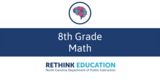Conditional Remix & Share Permitted
CC BY-NC-SA
Rating
0.0 stars

This course was created by the Rethink Education Content Development Team in partnership with the North Carolina Virtual Public Schools. This course is aligned to the NC Standards for 8th Grade Math.&nbsp;

Subject:
Mathematics
Material Type:
Activity/Lab
Assessment
Formative Assessment
Full Course
Homework/Assignment
Lecture Notes
Vocabulary
Author:
Kelly Rawlston
Letoria Lewis